# 手把手教你用R語言建立信用評分模型（一）

How to master a skill

Jump into the middle of things,  get your hands dirty,  fall flat on your face,  and then reach for the stars.

—— Ben Stein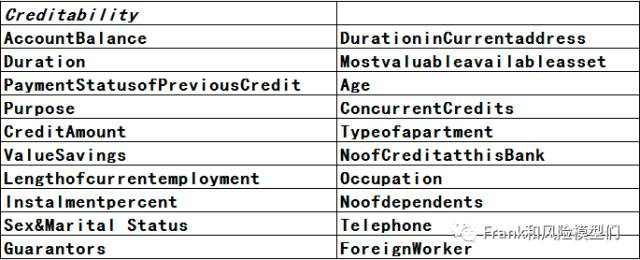library(caret)
train1 <-createDataPartition(y=german_credit\$Creditability,p=0.75,list=FALSE)
train <- german_credit[train1, ]
test <- german_credit[-train1, ]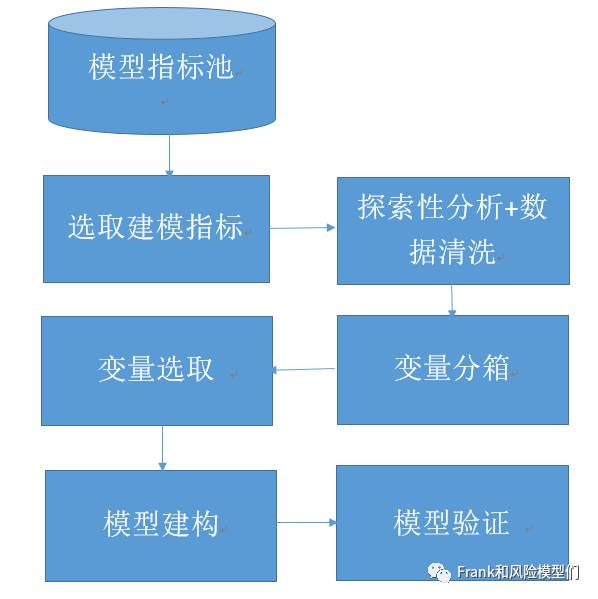require(caret)

data(GermanCredit)

ggplot(GermanCredit, aes(x = duration,y = ..count..,)) + geom_histogram(fill = “blue”, colour = “grey60”, size = 0.2, alpha = 0.2,binwidth = 5)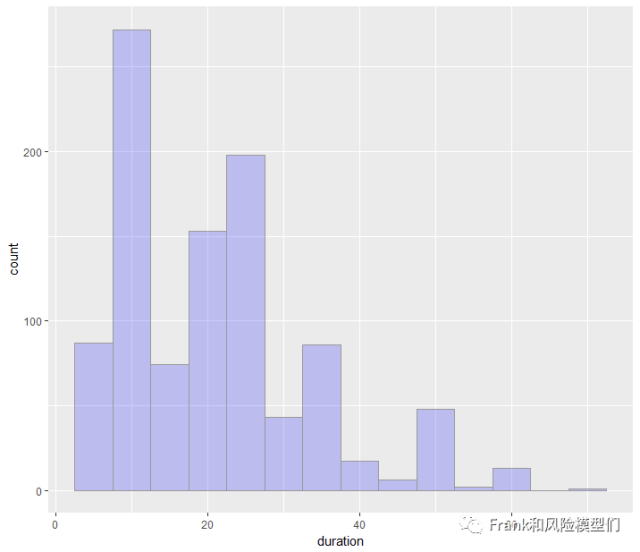ggplot(GermanCredit, aes(x = Amount,y = ..count..,)) + geom_histogram(fill = “blue”, colour = “grey60”, size = 0.2, alpha = 0.2,binwidth = 1000)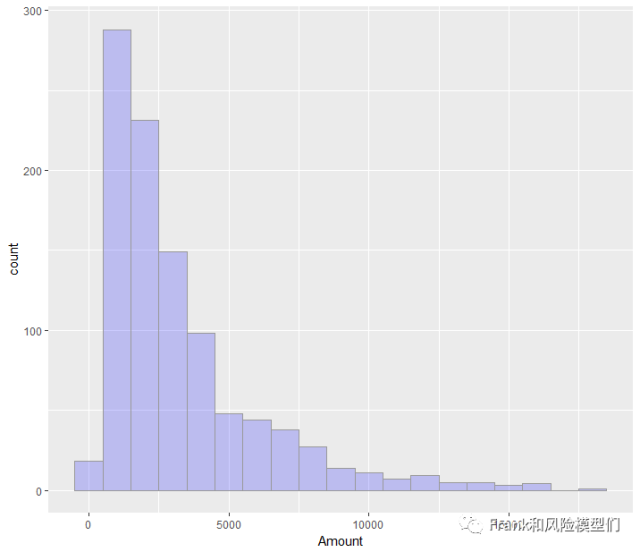ggplot(GermanCredit, aes(x =Creditability,y = ..count..,)) + geom_histogram(fill = “blue”, colour = “grey60″ , alpha = 0.2,stat=”count”)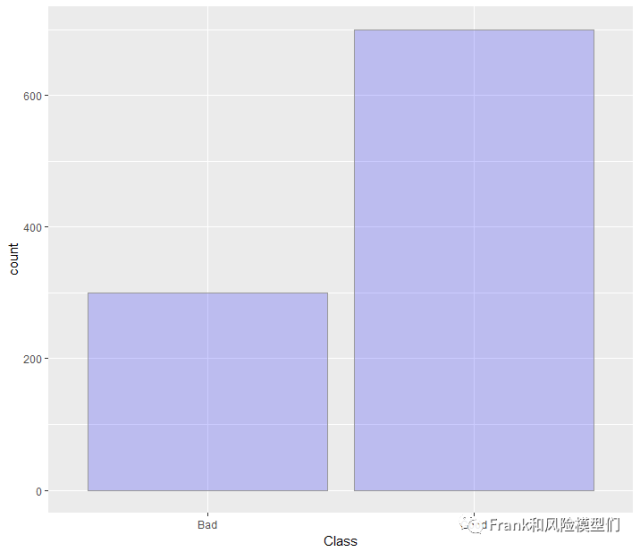library(smbinning)
Durationresult=smbinning(df=train,y=”Creditability”,x=”Duration”,p=0.05)
CreditAmountresult=smbinning(df=train2,y=”Creditability”,x=”CreditAmount”,p=0.05)
Ageresult=smbinning(df=train2,y=”Creditability”,x=”Age”,p=0.05)

smbinning.plot(CreditAmountresult,option=”WoE”,sub=”CreditAmount”)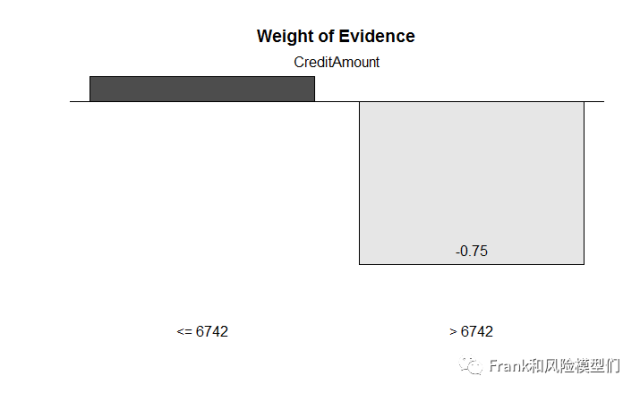smbinning.plot(Durationresult,option=”WoE”,sub=”Duration”)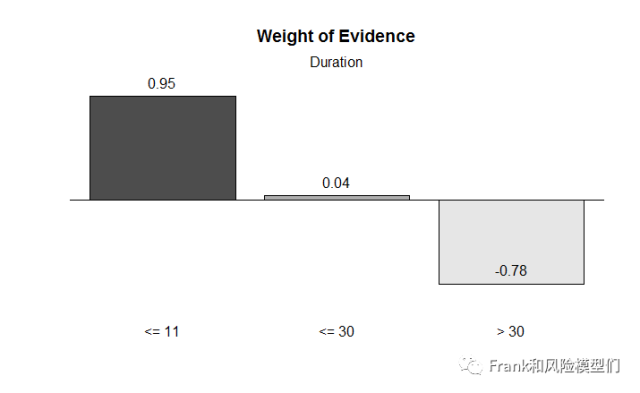smbinning.plot(Ageresult,option=”WoE”,sub=”Age”)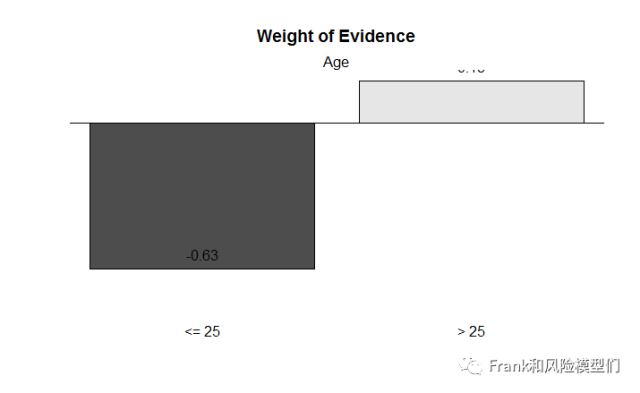未完待續，歡迎分享

未完待續，歡迎分享

Reference：

未完待續，歡迎分享1月原創好文回顧，請點擊閱讀：

7扇門｜人臉識別技術有漏洞？快來看看如何升級